Your data analysis solution

# Ordinal logit model in Excel tutorial

2017-10-20

This tutorial will help you set up and interpret an Ordinal Logit model in Excel using the XLSTAT software.
Not sure this is the modeling feature you are looking for? Check out this guide

## Ordinal logit model

The ordinal logit model is a specific logit model. It applies when the response variable has ordered categories. This method is very useful when one wants to understand, or to predict, the effect of a series of variables on an ordered qualitative response variable. Ordered logit model can be helpful to model the effect of some descriptive variables on the satisfaction with respect to a brand in a market.

With XLSTAT you can run the ordinal logit on raw data. The dialog box for to the ordinal logit model is the same as the one used for the logistic regression.

The methodology of ordinal logit model aims at modeling the cumulative probability associated to each category smaller or equal to the studied category depending on the values of the explanatory variables, which can be categorical or numerical variables.

## Dataset for running an ordinal logit model

The example treated here looks at factors that influence the decision of whether to apply to graduate school.  College juniors are asked if they are unlikely, somewhat likely, or very likely to apply to graduate school.  Hence, our outcome variable has three categories.  Data on parental educational status, whether the undergraduate institution is public or private, and current GPA is also collected. The researchers have reason to believe that the "distances" between these three points are not equal.  For example, the "distance" between "unlikely" and "somewhat likely" may be shorter than the distance between "somewhat likely" and "very likely".

An Excel sheet with both the data and the results can be downloaded by clicking here.

## Goal of this ordinal logit model

Our goal is to understand if students are likely to apply to graduate school.

## Setting up an ordinal logit model

To activate the Ordinal Logit Model dialog box, start XLSTAT, then select the XLSTAT / Modeling data / Logistic regression for binary response data command, or click on the logistic regression button of the Modeling Data toolbar (see below).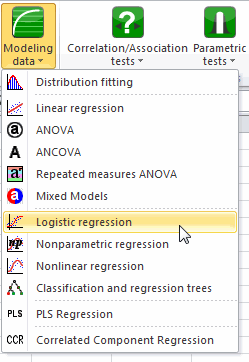When you click on the button, the Logistic regression dialog box appears.

To activate the ordinal logit model, change the response type and choose ordinal.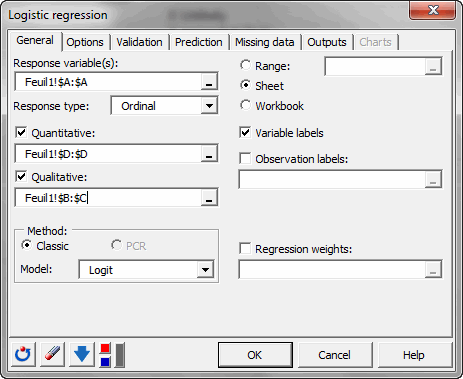Select the data on the Excel sheet. The Response corresponds to the column where the variable to be explained is stored. In this particular case we have two qualitative explanatory variables and one quantitative explanatory variable.

As we selected the column titles of all variables, we have selected the option Variable labels included.

Many other options are available in the dialog box.

Note: The default options correspond to the basic choice one would make; please consult the XLSTAT help for more details.

The computations begin once you have clicked on the OK button.

## Interpret the results of a ordinal logit model

The following table gives several indicators on the model quality (or goodness of fit). These results are equivalent to the R² and to the analysis of variance table in linear regression and ANOVA. The most important value to look at is the probability of the Chi-square test on the log ratio. This is equivalent to the Fisher's F test: we try to evaluate if the variables bring significant information by comparing the model as it is defined, with a simpler model with only one constant. In this case, as the probability is lower than 0.0001, we can conclude that significant information is brought by the variables.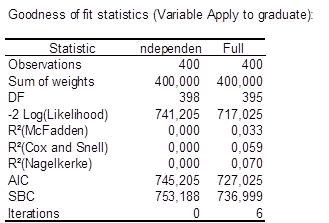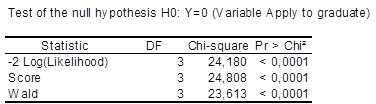The next table gives details on each explanatory variable of the model. It is a Type III table as in linear regression.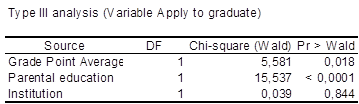We see that the grade point average and the parental education have a significant impact. Public/private institution has not a significant impact.

The next table gives details on the model. This table is helpful in understanding the effect of the various variables on the categories of the response variable. It is different from the logistic regression table. There is one intercept for each category of the response variable and one set of coefficients since the parallel curves hypothesis is supposed to be met.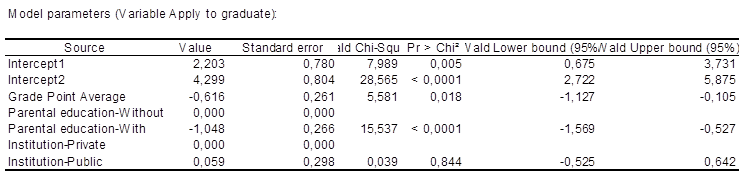Parameters interpretation is not immediate. The model equation for modality 1 (somewhat likely) is:

Log(P(Response<=1)/P(Response>1))=-2.203-0.616*GRADE-1.048*Parental Education-With+0.059*Institution-Public

For example, we can say that for one unit change in the variable GRADE, the log of the ratio of the two probabilities, P(Response<=1)/P(Response>1)), will be decreased by 0.616. Therefore, we can say that, in general, if you have a higher grade then the probability of saying that you are unlikely or somewhat likely to apply to graduate school will get smaller.

Other analysis are also possible using the individual probabilities or the classification tables.

### Contact Us

#### Contact our technical support team: support@xlstat.com

https://cdn.desk.com/
false
desk
Loading
seconds ago
a minute ago
minutes ago
an hour ago
hours ago
a day ago
days ago
about
false
Invalid characters found
/customer/portal/articles/autocomplete
9283